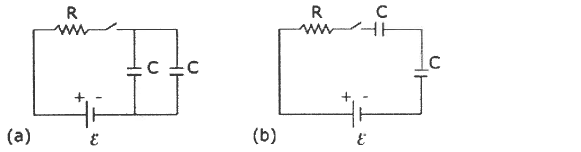# Problem: In which of the two circuits shown in the figure will the capacitors charge more slowly when the switch is closed?  (a) Circuit (a) (b) Circuit (b) (c) The capacitors will charge at the same rate in the two circuits.

###### FREE Expert Solution
90% (440 ratings)
###### Problem Details

In which of the two circuits shown in the figure will the capacitors charge more slowly when the switch is closed?

(a) Circuit (a)

(b) Circuit (b)

(c) The capacitors will charge at the same rate in the two circuits.Frequently Asked Questions

What scientific concept do you need to know in order to solve this problem?

Our tutors have indicated that to solve this problem you will need to apply the !! Resistor-Capacitor Circuits concept. If you need more !! Resistor-Capacitor Circuits practice, you can also practice !! Resistor-Capacitor Circuits practice problems.

How long does this problem take to solve?

Our expert Physics tutor, Douglas took 2 minutes and 47 seconds to solve this problem. You can follow their steps in the video explanation above.

What professor is this problem relevant for?

Based on our data, we think this problem is relevant for Professor Rodriguez's class at FIU.